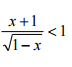# Inequality. What did I do wrong?

#### dukeham

##### New member
*English is not my native language. Sorry if i misspelled wrong.
Solve the inequality: (x+1)/sqrt(1-x)< 1I know that the answer is: (-∞,0) , but i can´t get to it

First: 1-x ≥ 0
x ≤ 1 (-∞,1]

Then I resolve the exercise:

-multiply both sides by sqrt(1-x) and simplify x+1< sqrt(1-x)
-square both sides and cancel out square root (x+1)^2 < 1-x
-expand binomial x^2+2x+1<1-x
-subtract 1-x from both sides and simplify x^2+3x<0
-factor and find signs -3<x<0
-Combine ranges x≤1 and -3<x<0

What did I do wrong?

#### Subhotosh Khan

##### Super Moderator
Staff member
*English is not my native language. Sorry if i misspelled wrong.
Solve the inequality: (x+1)/sqrt(1-x)< 1
View attachment 11743

I know that the answer is: (-∞,0) , but i can´t get to it

First: 1-x ≥ 0
x ≤ 1 (-∞,1]

Then I resolve the exercise:

-multiply both sides by sqrt(1-x) and simplify x+1< sqrt(1-x)
-square both sides and cancel out square root (x+1)^2 < 1-x
-expand binomial x^2+2x+1<1-x
-subtract 1-x from both sides and simplify x^2+3x<0
-factor and find signs -3<x<0
-Combine ranges x≤1 and -3<x<0

What did I do wrong?
-subtract 1-x from both sides and simplify x^2+3x<0

x(x+3) < 0 .......................... Two solutions

Either x>0 and (x+3)<0 ............................impossible

Or

x<0 and x+3>0

then x > -3 .................. which implies

-3 < x < 0

•topsquark

#### Dr.Peterson

##### Elite Member
Squaring both sides of an inequality does not preserve the inequality. Your error is in assuming it does.

I would either break it into cases before squaring, or just graph the two sides. Using cases, note that if both sides of an inequality are positive, then squaring will preserve it; for example, 1 < 2 becomes 1 < 4. But if the left side is negative while the right is positive, e.g. -3 < 2, then it is always true, and you don't need to do anything further. Squaring would have produced 9 < 4, which is false.

The solution is not (-3, 0); just check the inequality at, say, -3 and -4 to see this.

•topsquark

#### lookagain

##### Senior Member
Solve the inequality: (x+1)/sqrt(1-x)< 1
View attachment 11743

I know the answer is: (-∞,0)
.
.
.
$$\displaystyle \dfrac{x + 1}{\sqrt{1 - x}} \ - \ 1 < 0$$

$$\displaystyle \dfrac{x + 1 }{\sqrt{1 - x}} \ - \ \dfrac{\sqrt{1 - x}}{\sqrt{1 - x}} < 0$$

$$\displaystyle \dfrac{x + 1 \ - \ \sqrt{1 - x}}{\sqrt{1 - x}} < 0$$

For potential critical numbers, set $$\displaystyle \ \ (x + 1 \ - \ \sqrt{1 - x}) \ \$$ equal to 0, and set $$\displaystyle \ \ \sqrt{1 - x} \ \$$ equal to 0.

x = 0 and x = 3 are candidates for solutions to the first equation, but x = 3 is extraneous and must be discarded..
x = 1 is the solution to the second equation.

Looking at test values on a number line:

To the right of x = 1: The expression is undefined.

Between x = 0 and x = 1, you could choose a convenient x-value because of the square root, say x = 3/4. The sign of the
expression is positive.

x = -3 turned out not to be a critical number. And, because it is to the left of the remaining critical number x = 0, and it is
a convenient choice because of the square root, then you may substitute it and see that the sign of the expression is negative.

So, the solution for the problem is $$\displaystyle \$$ x < 0, or $$\displaystyle \ \ (-\infty, 0)$$.

•dukeham

#### pka

##### Elite Member
Here is a slightly different approach.
After reducing the inequality to $$\displaystyle \dfrac{(x + 1) \ - \ \sqrt{1 - x}}{\sqrt{1 - x}} < 0$$
note that only the numerator determines the sign. The radical forces $$\displaystyle x<1$$.

If $$\displaystyle x<0$$ then $$\displaystyle (x+1)<1~\&~\sqrt{1-x}>1$$ so the numerator is negative.

We know that $$\displaystyle x=0$$ does not work so that leaves $$\displaystyle a\in(0,1)$$ to consider.
But then we would have $$\displaystyle (a+1)>1~\&~\sqrt{1-a}<1$$ so the numerator is positive and we don't want that.

Hence we have a solution: $$\displaystyle x<0$$.

•Jomo and dukeham

#### dukeham

##### New member
Thank you all for the answers.